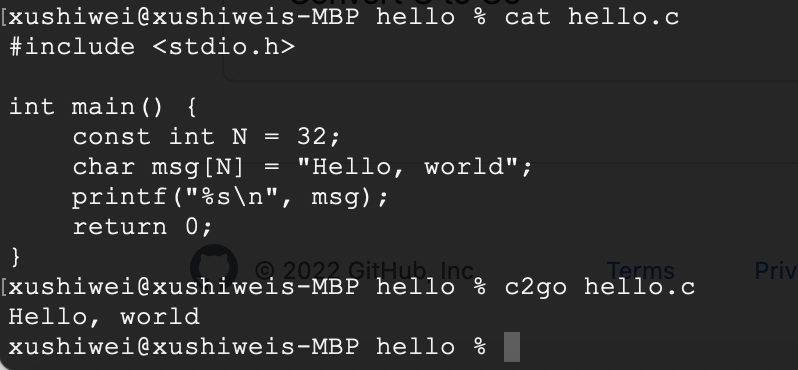Popularity
5.4
Growing
Activity
9.7
Declining
233
6
14

Programming language: Go
Tags: Go Compilers

# c2go alternatives and similar packages

Based on the "Go Compilers" category.
Alternatively, view c2go alternatives based on common mentions on social networks and blogs.

• ### gopherjs

A compiler from Go to JavaScript for running Go code in a browser
• ### llgo

LLVM-based compiler for Go
• ### Clean code begins in your IDE with SonarLint

Up your coding game and discover issues early. SonarLint is a free plugin that helps you find & fix bugs and security issues from the moment you start writing code. Install from your favorite IDE marketplace today.
• ### android-go

The android-go project provides a platform for writing native Android apps in Go programming language.
• ### tardisgo

Golang->Haxe->CPP/CSharp/Java/JavaScript transpiler
• ### c4go

Transpiling C code to Go code
• ### esp32

Transpile Golang into Arduino code to use fully automated testing at your IoT projects.
• ### f4go

Transpiling fortran code to golang code

Do you think we are missing an alternative of c2go or a related project?

## c2go - Convert C to Go

This is a subproject of the Go+ project. Its goal is converting any C project into Go without any human intervention and keeping performance close to C.## How to run examples?

Run an example:

• Build c2go tools: `go install -v ./...`
• Go `testdata/xxx` directory, and run `c2go .`

Run/Test multiple examples:

• Run examples: `c2go ./...`
• Test examples: `c2go -test ./...`

## How c2go is used in Go+

``````import "C"

C.printf C"Hello, c2go!\n"
C.fprintf C.stderr, C"Hi, %7.1f\n", 3.14
``````

Here we use `import "C"` to import libc. It's an abbreviation for `import "C/github.com/goplus/libc"`. It is equivalent to the following code:

``````import "C/github.com/goplus/libc"

C.printf C"Hello, c2go!\n"
C.fprintf C.stderr, C"Hi, %7.1f\n", 3.14
``````

In this example we call two C standard functions `printf` and `fprintf`, passing a C variable `stderr` and two C strings in the form of `C"xxx"` (a Go+ syntax to represent C style strings).

Run `gop run .` to see the output of this example:

``````Hello, c2go!
Hi,     3.1
``````

## What's our plan?

• First, support most of the syntax of C. Stage: `Done Almost`, see supported C syntax.
• Second, compile `sqlite3` to fix c2go bugs and get list of its dependent C standard libary fuctions. Stage: `Done`, see github.com/goplus/sqlite and its dependent fuctions.
• Third, support most of C standard library functions (especially that used by `sqlite3`), and can import them by Go+. Stage: `Doing`, see detailed progress here.
• Last, support all custom libraries, especially those well-known open source libraries. Stage: `Planning`.

## Tested Platform

• [x] MacOS: 1.17.x
• [x] Linux: ubuntu-20.04 (temporarily skip `testdata/qsort`)
• [ ] Windows

## Supported C syntax

### Data structures

• [x] Void: `void`
• [x] Boolean: `_Bool`, `bool`
• [x] Integer: [`signed`/`unsigned`] [`short`/`long`/`long long`] `int`
• [x] Enum: `enum`
• [x] Float: `float`, `double`, `long double`
• [x] Character: [`signed`/`unsigned`] `char`
• [ ] Wide Character: `wchar_t`
• [ ] Large Integer: [`signed`/`unsigned`] `__int128`
• [x] Complex: `_Complex` `float`/`double`/`long double`
• [x] Typedef: `typedef`
• [x] Pointer: *T, T[]
• [x] Array: T[N], T[]
• [x] Array Pointer: T(*)[N]
• [x] Function Pointer: T (*)(T1, T2, ...)
• [x] Struct: `struct`
• [x] Union: `union`
• [x] BitField: `intType :N`

### Operators

• [x] Arithmetic: a+b, a-b, a*b, a/b, a%b, -a, +a
• [x] Increment/Decrement: a++, a--, ++a, --a
• [x] Comparison: ab, a>=b, a==b, a!=b
• [x] Logical: a&&b, a||b, !a
• [x] Bitwise: a|b, a&b, ab, ~a, a<>n
• [x] Pointer Arithmetic: p+n, p-n, p-q, p++, p--
• [x] Assignment: `=`
• [x] Operator Assignment: a`<op>=`b
• [x] BitField Assignment: `=`
• [ ] BitField Operator Assignment: a`<op>=`b
• [x] Struct/Union/BitField Member: a.b
• [x] Array Member: a[n]
• [x] Pointer Member: &a, *p, p[n], n[p], p->b
• [x] Comma: `a,b`
• [x] Ternary Conditional: cond?a:b
• [x] Function Call: f(a1, a2, ...)
• [x] Conversion: (T)a
• [x] Sizeof: sizeof(T), sizeof(a)
• [x] Offsetof: __builtin_offsetof(T, member)

### Literals

• [x] Boolean, Integer
• [x] Float, Complex Imaginary
• [x] Character, String
• [ ] Array: `(T[]){ expr1, expr2, ... }`
• [ ] Array Pointer: `&(T[]){ expr1, expr2, ... }`
• [ ] Struct: `struct T{ expr1, expr2, ... }`

### Initialization

• [x] Basic: `T a = expr`
• [x] Array: `T a[] = { expr1, expr2, ... }`, `T a[N] = { expr1, expr2, ... }`
• [x] Struct: `struct T a = { expr1, expr2, ... }`, `struct T a = { .a = expr1, .b = expr2, ... }`
• [x] Union: `union T a = { expr }, union T a = { .a = expr }`
• [x] Array in Struct: `struct { T a[N]; ... } v = { { expr1, expr2, ... }, ... }`, `struct { T a[N]; ... } v = { { .a = expr1, .a = expr2, ... }, ... }`

### Control structures

• [x] If: `if (cond) stmt1 [else stmt2]`
• [x] Switch: `switch (tag) { case expr1: stmt1 case expr2: stmt2 default: stmtN }`
• [x] For: `for (init; cond; post) stmt`
• [x] While: `while (cond) stmt`
• [x] Do While: `do stmt while (cond)`
• [x] Break/Continue: `break`, `continue`
• [x] Goto: `goto label`

### Functions

• [x] Parameters